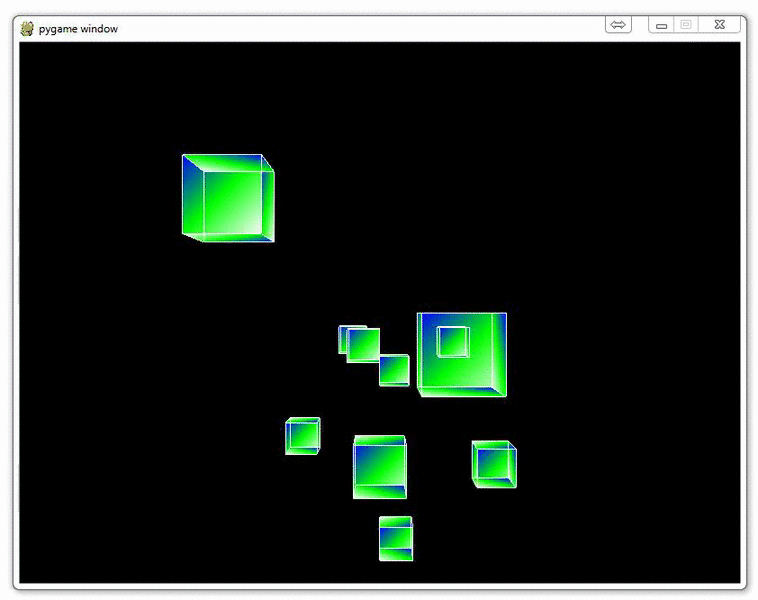## Adding Many Cubes to our Game

Now that we've made one random cube, why not make many? After all, we can probably see here that avoiding one cube on a plane with x,y and z is quite simple!

To create a bunch of random cubes, we're going to start off with a new function, set_vertices.

```def set_vertices(max_distance):
x_value_change = random.randrange(-10,10)
y_value_change = random.randrange(-10,10)
z_value_change = random.randrange(-1*max_distance,-20)
```

To start, we have a parameter, max_distance, which is what it sounds like. It is the maximum distance out that we're going to consider drawing cubes.

From there, we define some changes for x, y and z, which pertains to the changes we're going to make to our initial cube!

With that, the next bit to our function:

```    new_vertices = []

for vert in vertices:
new_vert = []

new_x = vert + x_value_change
new_y = vert + y_value_change
new_z = vert + z_value_change
```

So first we start off with an empty list, then we begin our for loop running through the vertices list.

Finally in this loop and concluding our function:

```        new_vert.append(new_x)
new_vert.append(new_y)
new_vert.append(new_z)

new_vertices.append(new_vert)

return new_vertices
```

We populate our new vertices list and then return the new set of cube vertices, ready to be rendered!

Now we just need to make a parameter change to our cube function:

```def Cube(vertices):

for surface in surfaces:
x = 0

for vertex in surface:
x+=1
glColor3fv(colors[x])
glVertex3fv(vertices[vertex])

glEnd()

glBegin(GL_LINES)
for edge in edges:
for vertex in edge:
glVertex3fv(vertices[vertex])
glEnd()
```

From there, in our main function, we want to change the while loop to a simple while True, then we need to add a max_distance variable, an empty cube dict, the a for loop for how many cubes we want.

```def main():
pygame.init()
display = (800,600)
pygame.display.set_mode(display, DOUBLEBUF|OPENGL)

gluPerspective(45, (display/display), 0.1, 50.0)

glTranslatef(random.randrange(-5,5),random.randrange(-5,5), -40)

#object_passed = False

x_move = 0
y_move = 0
# max_distance
max_distance = 100
# make as many cubes as in the range!
cube_dict = {}

for x in range(20):
cube_dict[x] =set_vertices(max_distance)

while True:
for event in pygame.event.get():
if event.type == pygame.QUIT:
pygame.quit()
quit()

if event.type == pygame.KEYDOWN:
if event.key == pygame.K_LEFT:
x_move = 0.3
if event.key == pygame.K_RIGHT:
x_move = -0.3

if event.key == pygame.K_UP:
y_move = -0.3
if event.key == pygame.K_DOWN:
y_move = 0.3

if event.type == pygame.KEYUP:
if event.key == pygame.K_LEFT or event.key == pygame.K_RIGHT:
x_move = 0

if event.key == pygame.K_UP or event.key == pygame.K_DOWN:
y_move = 0

x = glGetDoublev(GL_MODELVIEW_MATRIX)
#print(x)

camera_x = x
camera_y = x
camera_z = x

glClear(GL_COLOR_BUFFER_BIT|GL_DEPTH_BUFFER_BIT)

glTranslatef(x_move,y_move,.50)
# draw them all!
for each_cube in cube_dict:
Cube(cube_dict[each_cube])

pygame.display.flip()
pygame.time.wait(10)
```

That's a lot of little things, and it's easy to miss something either for you or for me writing this up, so here's the full code just in case!

```import pygame
from pygame.locals import *

from OpenGL.GL import *
from OpenGL.GLU import *

import random

vertices = (
(1, -1, -1),
(1, 1, -1),
(-1, 1, -1),
(-1, -1, -1),
(1, -1, 1),
(1, 1, 1),
(-1, -1, 1),
(-1, 1, 1)
)

edges = (
(0,1),
(0,3),
(0,4),
(2,1),
(2,3),
(2,7),
(6,3),
(6,4),
(6,7),
(5,1),
(5,4),
(5,7)
)

surfaces = (
(0,1,2,3),
(3,2,7,6),
(6,7,5,4),
(4,5,1,0),
(1,5,7,2),
(4,0,3,6)
)

colors = (
(1,0,0),
(0,1,0),
(0,0,1),
(0,1,0),
(1,1,1),
(0,1,1),
(1,0,0),
(0,1,0),
(0,0,1),
(1,0,0),
(1,1,1),
(0,1,1),
)

def set_vertices(max_distance):
x_value_change = random.randrange(-10,10)
y_value_change = random.randrange(-10,10)
z_value_change = random.randrange(-1*max_distance,-20)

new_vertices = []

for vert in vertices:
new_vert = []

new_x = vert + x_value_change
new_y = vert + y_value_change
new_z = vert + z_value_change

new_vert.append(new_x)
new_vert.append(new_y)
new_vert.append(new_z)

new_vertices.append(new_vert)

return new_vertices

def Cube(vertices):

for surface in surfaces:
x = 0

for vertex in surface:
x+=1
glColor3fv(colors[x])
glVertex3fv(vertices[vertex])

glEnd()

glBegin(GL_LINES)
for edge in edges:
for vertex in edge:
glVertex3fv(vertices[vertex])
glEnd()

def main():
pygame.init()
display = (800,600)
pygame.display.set_mode(display, DOUBLEBUF|OPENGL)

gluPerspective(45, (display/display), 0.1, 50.0)

glTranslatef(random.randrange(-5,5),random.randrange(-5,5), -40)

#object_passed = False

x_move = 0
y_move = 0

max_distance = 100

cube_dict = {}

for x in range(20):
cube_dict[x] =set_vertices(max_distance)

#glRotatef(25, 2, 1, 0)

while True:
for event in pygame.event.get():
if event.type == pygame.QUIT:
pygame.quit()
quit()

if event.type == pygame.KEYDOWN:
if event.key == pygame.K_LEFT:
x_move = 0.3
if event.key == pygame.K_RIGHT:
x_move = -0.3

if event.key == pygame.K_UP:
y_move = -0.3
if event.key == pygame.K_DOWN:
y_move = 0.3

if event.type == pygame.KEYUP:
if event.key == pygame.K_LEFT or event.key == pygame.K_RIGHT:
x_move = 0

if event.key == pygame.K_UP or event.key == pygame.K_DOWN:
y_move = 0

##            if event.type == pygame.MOUSEBUTTONDOWN:
##                if event.button == 4:
##                    glTranslatef(0,0,1.0)
##
##                if event.button == 5:
##                    glTranslatef(0,0,-1.0)

#glRotatef(1, 3, 1, 1)

x = glGetDoublev(GL_MODELVIEW_MATRIX)
#print(x)

camera_x = x
camera_y = x
camera_z = x

glClear(GL_COLOR_BUFFER_BIT|GL_DEPTH_BUFFER_BIT)

glTranslatef(x_move,y_move,.50)

for each_cube in cube_dict:
Cube(cube_dict[each_cube])

pygame.display.flip()
pygame.time.wait(10)

main()
pygame.quit()
quit()

```

The next tutorial:• OpenGL with PyOpenGL introduction and creation of Rotating Cube

• Coloring Surfaces as well as understand some of the basic OpenGL code

• Understanding navigation within the 3D environment via OpenGL

• Moving the player automatically towards the cube

• Random Cube Position

• Adding Many Cubes to our Game
• Adding a ground in OpenGL

• Infinite flying cubes

• Optimizing the processing for infinite cubes